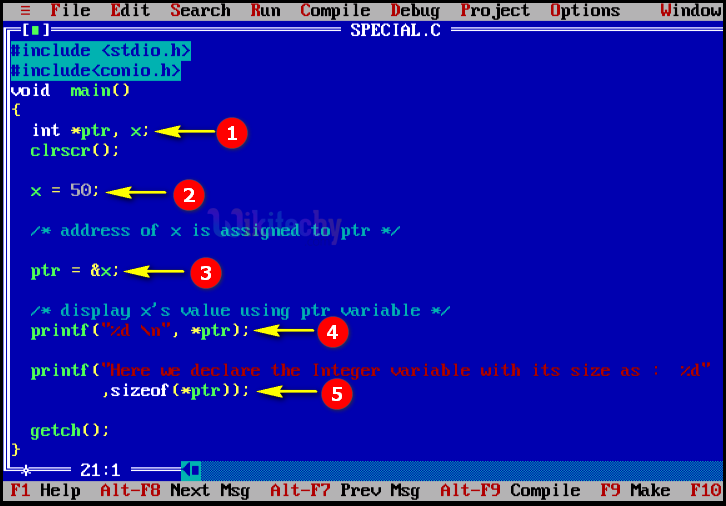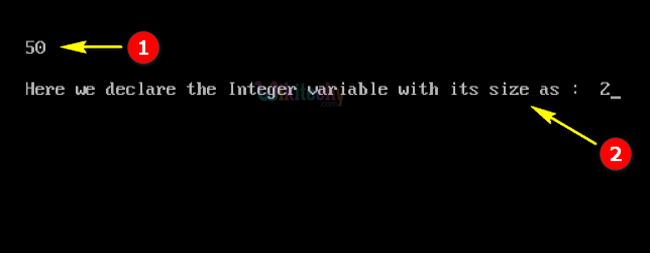# Special Operators in C ProgrammingLearn C - C tutorial - Special operators in c programming - C examples - C programs

## Special Operators in C Programming - Definition and Usage

• In C Programming the special operators are mostly used for memory related functions.
• In C-language some special operators are listed below as follows:
• & Operator is used to get the address of the variable.The real address or physical address of a variable is the real location of the variable in memory and is usually only known to the OS.
• * Operator is used as pointer it stores/points the address of another variable.It is used to allocate memory dynamically.Pointer variable might be belonging to any of the data type such as int, float, char, double, short etc.of the variable.
• Sizeof() Operator is a keyword, but it is a compile-time operator that determines the size, in bytes, of a variable or data type.It is used to get the size of classes, structures, unions and any other user defined data type.

Primitive data Types.## C Code - Explanation1. In this statement we have declared the variable “x” as integer and *ptr as pointer variable .
2. Here we are assigning the value of the variable “x” as “50”.
3. In this statement the ptr variable holds the address of “x”.
4. In this, printf statement is used for displaying the value of the variable “*ptr” in your console output window.
5. In this print statement we are printing the size of the datatype value and also here we are defining the variable *ptr which have the value as integer variable where the variable size as “2”

Operator in C

## Sample Output - Programming Examples1. Here in this output the value of the *ptr variable “50” has been displayed and printed in the console window.
2. Here in this output the size of integer datatype “2” of the variable has printed and displayed with its print statement “Here we declare the Integer variable with its size as: 2”